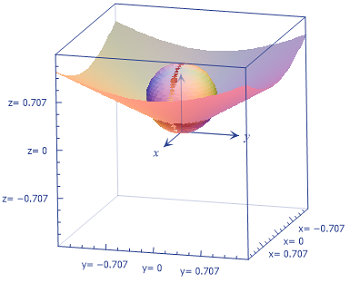Consider the solid bounded by the sphere x^2+y^2+z^2=z that is above the cone z=1 sqrt3 ...

Question:

Consider the solid bounded by the sphere {eq}\displaystyle x^2+y^2+z^2=z {/eq} that is above the cone {eq}\displaystyle z=\frac{1}{\sqrt{3}}\sqrt{x^2+y^2}. {/eq} Sketch the solid and use spherical coordinates to find the volume of the solid.

Spherical Coordinates:

Let's face it: spherical coordinates make most people cringe when they see them. All those Greek letters and trig functions can be intimidating. But for the most part, it's really not so bad, especially if they are use efficiently. Recall

{eq}x=\rho \cos \theta \sin \phi {/eq}

{eq}y = \rho \sin \theta \sin \phi {/eq}

{eq}z = \rho \cos \phi {/eq}

{eq}\rho^2 = x^2+y^2+z^2 {/eq}

{eq}dV = \rho^2 \sin \phi \ d\rho \ d\phi \ d\theta {/eq}

Become a Study.com member to unlock this answer! Create your account

Let's look at the region first:Note that we are dealing with that region above the cone and inside the sphere. Let's get these surfaces into a...Cylindrical & Spherical Coordinates: Definition, Equations & Examples

from

Chapter 13 / Lesson 10
21K

Cylindrical coordinates and spherical coordinates are similar to the cartesian coordinate system but applied to specifying points in a three-dimensional plane. Learn how polar coordinates apply to these calculations and their use in celestial maps.